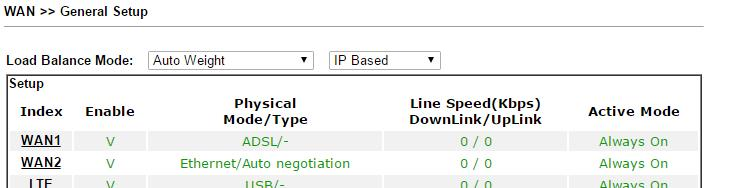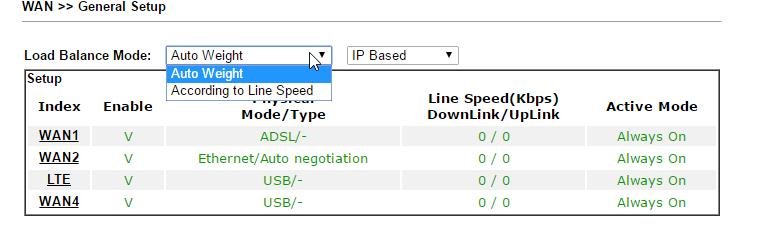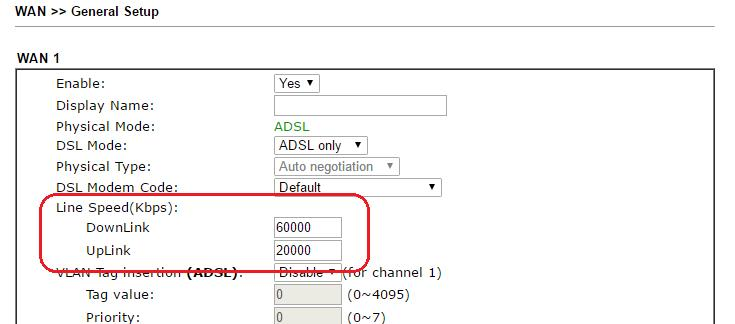What's the difference between "Auto Weight" and "According to Line Speed" Load Balance mode?

This article explains how does the default load balance work, and what's the difference between "Auto Weight" and "According to Line Speed" mode.How does the default load balance works?

For multi-WAN models, by default, Vigor Router will balance the traffic load across all the online WAN interfaces. The routing decision was made according to the ratio of each WAN's remaining bandwidth, which is calculated by subtracting the bandwidth currently used from the maximum bandwidth.

For example, suppose there are two WAN interfaces online, WAN1 and WAN2, WAN1's maximum bandwidth is 10Mpbs, and WAN2 is 20Mbps. If both WAN have 5Mbps traffic at the moment, then the remaining bandwidth of WAN1 is 5Mbps, and WAN2 is 15Mbps. The ratio of the remaining bandwidth of WAN1 to WAN2 is 1:3, so the load balance weight of WAN1 and WAN2 will be 1:3, which means if now there are four new sessions, one of them will be sent to WAN1, and three of them will be sent to WAN2.

And the router will keep monitoring the bandwidth used on each WAN interface, and update the load balance weight periodically.

What's the difference between "Auto Weight" and "According to Line Speed"?

On WAN >> General Setup page, there are two options for Load Balance Mode: Auto Weight and According to Line Speed. The difference between them is how does the router determine the "maximum bandwidth" of each WAN interface.The default setting is "Auto Weight". In this mode, the maximum bandwidth is defined according to the bandwidth peak that the WAN interface has reached recently. In "According to Line Speed" mode, the maximum bandwidth is the Line Speed value defined by the network administrator on WAN >> General Setup >> WAN index page. (Therefore, if you'd like to use According to Line Speed mode, don't forget to set the Line Speed for each WAN.)In summary, if the WAN interface has a dynamic line speed, using Auto Weight mode will allow the router to adjust the load balance weight according to the line status; however, using According to Line Speed mode will make sure the router won't underestimate the available bandwidth.